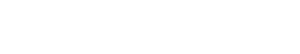# Dimensions

Dimensions refers to the number of distinct values that a particular variable can take on. For example, a variable such as “text length” would have a dimension of 1, while a variable such as “number of words” would have a dimension of 2.

Dimensions is often used interchangeably with other terms, such as “attributes” or “features”. However, there are some important distinctions between these terms. First, attributes are typically used to refer to nominal variables, while features are typically used to refer to ordinal or interval variables. Second, attributes can be thought of as the specific values that a variable can take on (e.g., “red” or “blue”), while features can be thought of as the characteristics of a variable that can be used to distinguish it from other variables (e.g., “length” or “width”).

So, when should you use the term Dimensions? If you are working with a nominal variable, then you may want to use the term “attributes”. If you are working with an ordinal or interval variable, then you may want to use the term “features”. However, if you are simply referring to the number of distinct values that a particular variable can take on, then the term “Dimensions” is likely to be the most appropriate.

## Measuring Dimensions

There are a few different ways to measure the dimension of a variable. The most common way is to simply count the number of distinct values that the variable can take on. For example, if a variable can take on the values 1, 2, 3, and 4, then it has a dimension of 4.

Another way to measure dimension is to use the concept of entropy. Entropy is a measure of the amount of information that is contained in a signal. In the context of dimension, entropy can be thought of as a measure of the number of bits required to encode the values of a variable. For example, if a variable can take on the values 1, 2, 3, and 4, then it would require 2 bits to encode the values.

The entropy of a variable can be calculated using the following formula:

Entropy = -sum(p_i * log2(p_i))

where p_i is the probability of the i-th value.

Using the formula above, we can see that the entropy of a variable with 4 values is 2 bits.

## Comparing Dimensions

When comparing the dimensions of two variables, it is important to keep in mind the different ways in which the dimension can be measured. For example, if we compare the dimension of a variable with 4 values to the dimension of a variable with 8 values, the variable with 8 values will have a greater dimension if we are measuring by entropy.

However, if we are simply counting the number of distinct values, then the variable with 4 values will have a greater dimension.

So, when comparing dimensions, it is important to be clear about which measure you are using.Unlock the power of actionable insights with AI-based natural language processing.